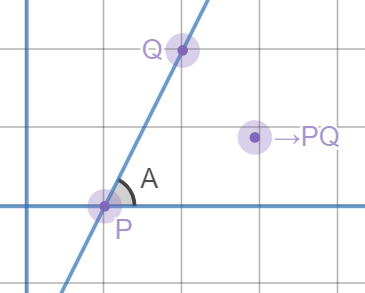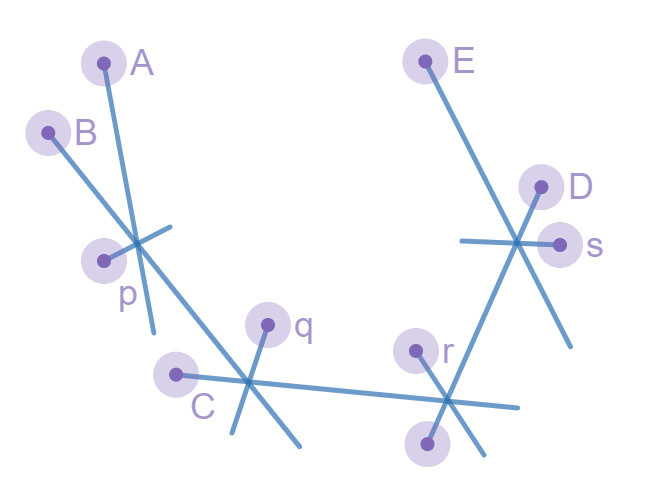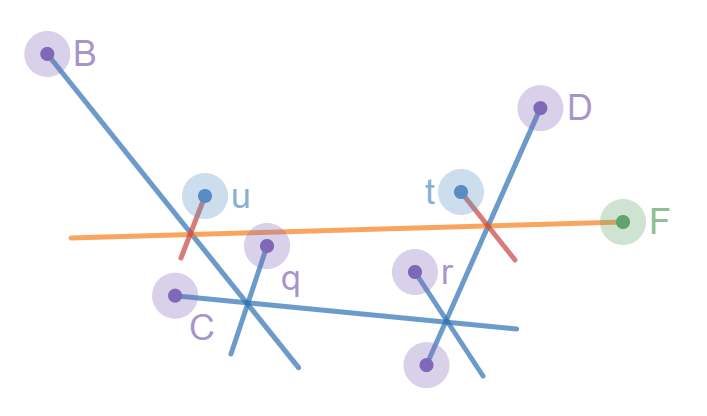×

Search anything:

# Halfplane Intersection problem

#### computational geometry AlgorithmsGet this book -> Problems on Array: For Interviews and Competitive Programming

• Introduction
• Brute Force
• Incrementatal method
• Sorting and increment algorithm
• Applications
• Overview

# Introduction

In this article, we will be discussing how to compute the intersection of a set of halfplanes. A half plane can be defined as a planar region which consists of all points on one side of an infinite straight line, and none on the other side. We can apply computational geometry here, as an intersection can be represented as a convex polygon, where every point inside it is also inside all halfplanes. If we can construct this polygon, we can retrieve the intersections.

We can state that N is the number of halfplanes in our set. Representing lines and halfplanes by a point and a vector, or in other terms, any point that lies on said line and the direction vector of the line. Each halfplane allows the region to the left of its direction vector. We can define the angle of a given half plane as the polar coordinates of its direction vector. We can also state that a resultant intersection is always either bounded or empty, in cases where this doesn't hold, we can add 4 more halfplanes which increase the size of the bounding box. For now we shall also assume that parallel halfplanes will not be present. To get a more visual representation of this, see the image below:This halfplne has an equation y >= 2x-2 and can be reprented as P = (1,0) with the direction vector PQ as (1,2).

I will now describe various ways of solving this problem, explaining algorithms in increasing efficiency.

# Brute Force

This problem can be solved using brute force through computing the intersection point of the lines and all pairs of halfplanes then, for every point, check if it is inside all of the other halfplanes. There are O(N^2) intersection points which need to be checked against O(N) halfplanes, resulting in the final complexity being O(N^3). We can then create a convex hull on the set of intersection points which were also included in the halfplanes. The vertices of this convex hull are the intersection points of the halfplane lines, as they of course that would mean they are points in every half plane since it is points of intersection. This approach works to gain a solution however is impractical to apply in a real world scenario due to the extremely poor efficiency.

# Incremental

A slightly faster approach to this problem would be to incrementally construct the intersection of the halfplanes one by one. We essentially cut the convex polygon by a line N amount of times, whilst removing the redundant planes each time.

If we represent the convex polygon as a set of line segments, we can cut it with a halfplane by finding the points of intersection of the segments with the halfplane line, replacing all the line segments in the middle with a new segment corresponding to a halfplane. This can be implemented in O(N) time, and decrease the bounding box with each halfplane, resulting in an O(N^2) complexity.

This is much better than our initial approach however still relatively inefficient as we still have to loop over O(N) halfplanes with every iteration, we shall make some alterations to this algorithm in the next approach to recieve a reasonable solution.

# Sort and Increment Algorithm

We can use some properties of the output of the previous algorithm to optimise it. we know that the resulting region of the intersection of halfplanes is convex, therefore it will consist of some segments of halfplanes in order of their angles. Meaning, if we incrementally intersect the halfplanes in the order of their angles, storing them in a double-ended queue, we will only actually need to remove halfplanes from the front and the back of the queue.

Let's say that the halfplane angles are sorted from -π to π, and that we are about to start the kth step of the algorithm. We can say that we have already constructed the interesection of the halfplanes up to k-1. Because the halfplanes are sorted by angle, we can be sure that k will form a convex turn with the k-1 halfplane. From this we can say:

• Some of the halfplanes that are located at the back of the intersection may become redundant, in which case we shall pop from the queue from the back of the double-ended queue.
• Some of the halfplanes that are located at the front of the intersection may become redundant, in which case we shall pop from the queue from the back of the double-ended queue.
• The intersection may become empty from this, in this case we shall simply terminate the algorithm and return the intersection as empty.

In this case redundant means that it doesn't change the intersection or, the halfplane could be removed and the intersection wouldn't change.

To visualise this we shall let H = A,B,C,D,E. This is the set of halfplanes that are currently present in the intersection, for the points, we can define this as P = p,q,r,s which are intersection points of adjacent halfplanes from H. This could be represent as such below.Say we want to intersect it with a new halfplane F, it may make some existing halfplanes redundant.We can see F here represented in orange, it actually makes the halfplanes A and E redundant, therefore we can remove them from the front and back of the queue and add F at the end, then obtain a new intersection between H = B,C,D,F with P = q,r,t,u. This can be shown below:This is the main principle of the algorithm however there are a few special cases we should cover. In the case of parallel halfplanes there are 2 possible scenarios, either two that are parallel with the same direction or different directions. We should approach parallel lines with different directions the same as if we have to increase the size of the bounding box, since there will have to be at least one of the half planes within the bounding box in between the two since they are sorted by angle. This means that the only case we have to deal with is where there are two halfplanes with the same angle. To do this is straightforwards, simply keep the leftmost halfplane and era the rest since the others will eventaully become redundant anyway.

So, as an overview of the algorithm:

• Sort the set of halfplanes by angle, takes O(NlogN) time
• Iterate over the set, for each, perform incrementation and pop either from the front or back of the double-ended queue as necessary, takes linear time since every halfplane can only be added or removed once.
• Once this has completed, the convex polygon that results from the intersection can be obtained by computing the intersection points of adjacent halfplanes in the double-ended queue. It will take linear time. This results in our final complexity as O(NlogN). In a special case where the halfplanes are pre-sorted, this algorithm could be completed in O(N) however for the majority of cases we will be looking at O(NlogN), most of which is coming from the sorting by angle.

# Implementation

Here is an implementation of such an algorithm in code:

``````const long double eps = 1e-9, inf = 1e9;

struct Point {

long double x, y;
explicit Point(long double x = 0, long double y = 0) : x(x), y(y) {}

friend Point operator + (const Point& p, const Point& q) {
return Point(p.x + q.x, p.y + q.y);
}

friend Point operator - (const Point& p, const Point& q) {
return Point(p.x - q.x, p.y - q.y);
}

friend Point operator * (const Point& p, const long double& k) {
return Point(p.x * k, p.y * k);
}

friend long double cross(const Point& p, const Point& q) {
return p.x * q.y - p.y * q.x;
}
};

struct Halfplane {

Point p, pq;
long double angle;

Halfplane() {}
Halfplane(const Point& a, const Point& b) : p(a), pq(b - a) {
angle = atan2l(pq.y, pq.x);
}

bool out(const Point& r) {
return cross(pq, r - p) < -eps;
}

bool operator < (const Halfplane& e) const {
if (fabsl(angle - e.angle) < eps) return cross(pq, e.p - p) < 0;
return angle < e.angle;
}

bool operator == (const Halfplane& e) const {
return fabsl(angle - e.angle) < eps;
}

friend Point inter(const Halfplane& s, const Halfplane& t) {
long double alpha = cross((t.p - s.p), t.pq) / cross(s.pq, t.pq);
return s.p + (s.pq * alpha);
}
};

// Actual algorithm
vector<Point> hp_intersect(vector<Halfplane>& H) {

Point box = {
Point(inf, inf),
Point(-inf, inf),
Point(-inf, -inf),
Point(inf, -inf)
};

for(int i = 0; i<4; i++) {
Halfplane aux(box[i], box[(i+1) % 4]);
H.push_back(aux);
}

sort(H.begin(), H.end());
H.erase(unique(H.begin(), H.end()), H.end());

deque<Halfplane> dq;
int len = 0;
for(int i = 0; i < int(H.size()); i++) {

while (len > 1 && H[i].out(inter(dq[len-1], dq[len-2]))) {
dq.pop_back();
--len;
}

while (len > 1 && H[i].out(inter(dq, dq))) {
dq.pop_front();
--len;
}

dq.push_back(H[i]);
++len;
}

while (len > 2 && dq.out(inter(dq[len-1], dq[len-2]))) {
dq.pop_back();
--len;
}

while (len > 2 && dq[len-1].out(inter(dq, dq))) {
dq.pop_front();
--len;
}

if (len < 3) return vector<Point>();

vector<Point> ret(len);
for(int i = 0; i+1 < len; i++) {
ret[i] = inter(dq[i], dq[i+1]);
}
ret.back() = inter(dq[len-1], dq);
return ret;
}
``````

# Applications

The algorithm has many uses such as in visibility, we can use halfplane intersection to determine if some line segments are visible from some points within a plane, this could be used in video survelances, such as determining camera placement. Another applcation would be by checking if a solution exists to a set of linear constraints within linear programming. The linear constraints for two variables can be expreseed in the form Ax+By+C<=0, this can be reduced to half planes and therefore can be used in checking for feasible solutions. The intersections of the halfplanes represent the region of feasible solutions and then can be iteratively min/max'd by a linear function f(x,y).

# Overview

In this article at OpenGenus, we have detailed how to solve the problem of finding the intersection of a set of halfplanes by using computational geometry. We have present three different solutions, each one getting more refined. For each, the time complexity has been derived and each step has been clearly explained with relavent diagrams when needed. Our final approach was able to solve this with a average time complexity of O(NlogN) and O(N) in best case.